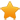Switch Editions?
CancelChannel: CodeSection,代码区,网络安全 - CodeSec
Viewing all articles

# 【技术分享】Lua程序逆向之Luac字节码与反汇编

0
0
【技术分享】Lua程序逆向之Luac字节码与反汇编

2017-11-17 15:00:25

【技术分享】Lua程序逆向之Luac文件格式分析

Luac指令在Lua中使用Instruction来表示，是一个32位大小的数值。在Luac.bt中，我们将其定义了为Inst结构体，回顾一下它的定义与读取函数：

typedefstruct(intpc){ localintpc_=pc; localucharinst_sz=get_inst_sz(); if(inst_sz==4){ uint32inst; }else{ Warning("Errorsize_Instruction."); } }Inst<optimize=false>;

Luac指令完整由：OpCode、OpMode操作模式，以及不同模式下使用的不同的操作数组成。

enumOpMode{iABC,iABx,iAsBx,iAx};

ABC这些字节大小与起始位置的定义如下：

#defineSIZE_C9 #defineSIZE_B9 #defineSIZE_Bx(SIZE_C+SIZE_B) #defineSIZE_A8 #defineSIZE_Ax(SIZE_C+SIZE_B+SIZE_A) #defineSIZE_OP6 #definePOS_OP0 #definePOS_A(POS_OP+SIZE_OP) #definePOS_C(POS_A+SIZE_A) #definePOS_B(POS_C+SIZE_C) #definePOS_BxPOS_C #definePOS_AxPOS_A

typedefenum{ /*---------------------------------------------------------------------- nameargsdescription ------------------------------------------------------------------------*/ OP_MOVE,/*ABR(A):=R(B)*/ OP_LOADK,/*ABxR(A):=Kst(Bx)*/ OP_LOADBOOL,/*ABCR(A):=(Bool)B;if(C)pc++*/ OP_LOADNIL,/*ABR(A):=...:=R(B):=nil*/ OP_GETUPVAL,/*ABR(A):=UpValue*/ OP_GETGLOBAL,/*ABxR(A):=Gbl[Kst(Bx)]*/ OP_GETTABLE,/*ABCR(A):=R(B)[RK(C)]*/ OP_SETGLOBAL,/*ABxGbl[Kst(Bx)]:=R(A)*/ OP_SETUPVAL,/*ABUpValue:=R(A)*/ OP_SETTABLE,/*ABCR(A)[RK(B)]:=RK(C)*/ ...... OP_CLOSE,/*Acloseallvariablesinthestackupto(>=)R(A)*/ OP_CLOSURE,/*ABxR(A):=closure(KPROTO[Bx],R(A),...,R(A+n))*/ OP_VARARG/*ABR(A),R(A+1),...,R(A+B-1)=vararg*/ }OpCode;

OpCode定义的注释中，详细说明了每一条指令的格式、使用的参数，以及它的含义。以第一条OP_MOVE指令为例，它接受两个参数R(A)与R(B)，的作用是完成一个赋值操作“R(A) := R(B)”。

LUAI_DDEFconstlu_byteluaP_opmodes[NUM_OPCODES]={ /*TABCmodeopcode*/ opmode(0,1,OpArgR,OpArgN,iABC)/*OP_MOVE*/ ,opmode(0,1,OpArgK,OpArgN,iABx)/*OP_LOADK*/ ,opmode(0,1,OpArgN,OpArgN,iABx)/*OP_LOADKX*/ ,opmode(0,1,OpArgU,OpArgU,iABC)/*OP_LOADBOOL*/ ,opmode(0,1,OpArgU,OpArgN,iABC)/*OP_LOADNIL*/ ,opmode(0,1,OpArgU,OpArgN,iABC)/*OP_GETUPVAL*/ ,opmode(0,1,OpArgU,OpArgK,iABC)/*OP_GETTABUP*/ ,opmode(0,1,OpArgR,OpArgK,iABC)/*OP_GETTABLE*/ ,opmode(0,0,OpArgK,OpArgK,iABC)/*OP_SETTABUP*/ ,opmode(0,0,OpArgU,OpArgN,iABC)/*OP_SETUPVAL*/ ,opmode(0,0,OpArgK,OpArgK,iABC)/*OP_SETTABLE*/ ,opmode(0,1,OpArgU,OpArgU,iABC)/*OP_NEWTABLE*/ ,opmode(0,1,OpArgR,OpArgK,iABC)/*OP_SELF*/ ,opmode(0,1,OpArgK,OpArgK,iABC)/*OP_ADD*/ ,opmode(0,1,OpArgK,OpArgK,iABC)/*OP_SUB*/ ...... ,opmode(0,1,OpArgU,OpArgN,iABx)/*OP_CLOSURE*/ ,opmode(0,1,OpArgU,OpArgN,iABC)/*OP_VARARG*/ ,opmode(0,0,OpArgU,OpArgU,iAx)/*OP_EXTRAARG*/ };

#defineopmode(t,a,b,c,m)(((t)<<7)|((a)<<6)|((b)<<4)|((c)<<2)|(m))

m位，占最低2位，即前面OpMode中定义的四种模式，通过它，可以确定OpCode的参数部分。

enumOpArgMask{ OpArgN,/*参数未被使用*/ OpArgU,/*已使用参数*/ OpArgR,/*参数是寄存器或跳转偏移*/ OpArgK/*参数是常量或寄存器常量*/ };

a位，占位6。表示是否是寄存器操作。

t位，占位7。表示是否是测试操作。跳转和测试指令该位为1。

printf("opcodever5.2:\n"); for(inti=0;i<sizeof(luaP_opmodes);i++){ printf("0x%x,",luaP_opmodes[i]); } printf("\n");

opcodever5.2: 0x60,0x71,0x41,0x54,0x50,0x50,0x5c,0x6c,0x3c,0x10,0x3c,0x54,0x6c,0x7c,0x7c,0x7c,0x7c,0x7c,0x7c,0x60,0x60,0x60,0x68,0x22,0xbc,0xbc,0xbc,0x84,0xe4,0x54,0x54,0x10,0x62,0x62,0x4,0x62,0x14,0x51,0x50,0x17,

OpCode获取

ucharGET_OPCODE(uint32inst){ return((inst)>>POS_OP)&((~((~(Instruction)0)<<(SIZE_OP)))<<(0)); }

#defineGETARG_A(i)getarg(i,POS_A,SIZE_A) #defineGETARG_B(i)getarg(i,POS_B,SIZE_B) #defineGETARG_C(i)getarg(i,POS_C,SIZE_C) #defineGETARG_Bx(i)getarg(i,POS_Bx,SIZE_Bx) #defineGETARG_Ax(i)getarg(i,POS_Ax,SIZE_Ax) #defineGETARG_sBx(i)(GETARG_Bx(i)-MAXARG_sBx)

intGETARG_A(uint32inst){ return((inst)>>POS_A)&((~((~(Instruction)0)<<(SIZE_A)))<<(0)); } intGETARG_B(uint32inst){ return((inst)>>POS_B)&((~((~(Instruction)0)<<(SIZE_B)))<<(0)); } intGETARG_C(uint32inst){ return((inst)>>POS_C)&((~((~(Instruction)0)<<(SIZE_C)))<<(0)); } intGETARG_Bx(uint32inst){ return((inst)>>POS_Bx)&((~((~(Instruction)0)<<(SIZE_Bx)))<<(0)); } intGETARG_Ax(uint32inst){ return((inst)>>POS_Ax)&((~((~(Instruction)0)<<(SIZE_Ax)))<<(0)); } intGETARG_sBx(uint32inst){ returnGETARG_Bx(inst)-MAXARG_sBx; }

//luadec_disassemble()fromluadecdisassemble.c stringInstructionRead(Inst&inst){ localinti=inst.inst; OpCodeo=(OpCode)GET_OPCODE(i); /* Printf("inst:0x%x\n",o); */ localinta=GETARG_A(i); localintb=GETARG_B(i); localintc=GETARG_C(i); localintbc=GETARG_Bx(i); localintsbc=GETARG_sBx(i); localintdest; localstringline; localstringlend; localstringtmpconstant1; localstringtmpconstant2; localstringtmp; localstringtmp2; localucharlua_version_num=get_lua_version(); localintpc=inst.pc_; //Printf("Inst:%s\n",EnumToString(o)); switch(o){ caseOP_MOVE: /* AB R(A):=R(B) */ SPrintf(line,"R%dR%d",a,b); SPrintf(lend,"R%d:=R%d",a,b); break; caseOP_LOADK://FIXMEOP_LOADKDecompileConstant /* ABx R(A):=Kst(Bx) */ SPrintf(line,"R%dK%d",a,bc); //Printf("OP_LOADKbc:%d\n",bc); tmpconstant1=DecompileConstant(parentof(parentof(inst)),bc); SPrintf(lend,"R%d:=%s",a,tmpconstant1); break; ...... caseOP_CLOSURE: { /* ABx R(A):=closure(KPROTO[Bx]) */ SPrintf(line,"R%d%d",a,bc); SPrintf(lend,"R%d:=closure(Function#%d)",a,bc); break; } default: break; } localstringss; SPrintf(ss,"[%d]%-9s%-13s;%s\n",pc,get_opcode_str(o),line,lend); returnss; } 上面的代码中，通过GET_OPCODE获取OpCode后，分别对它进行判断与处理，参数信息在函数的最开始获取，方便指令中使用。pc表示当前执行的指令所在位置，方便代码中做语义转换与依赖处理。代码中这一行需要注意：
DecompileConstant(parentof(parentof(inst))

typedefstruct(stringlevel){ localstringlevel_=level; //Printf("level:%s\n",level_); //header ProtoHeaderheader; //code //Codecode; structCode{ uint32sizecode<format=hex>; localucharinst_sz=get_inst_sz(); localintpc=1; if(inst_sz==4){ localuint32sz=sizecode; while(sz-->0){ Instinst(pc); pc++; } }else{ Warning("Errorsize_Instruction."); } typedefstruct(intpc){ localintpc_=pc; localucharinst_sz=get_inst_sz(); if(inst_sz==4){ uint32inst; }else{ Warning("Errorsize_Instruction."); } }Inst<read=InstructionRead,optimize=false>; }code<optimize=false>; ...... //upvaluenames UpValueNamesnames; }Proto<read=ProtoRead>;

stringget_opcode_str(OpCodeo){ stringstr=EnumToString(o); str=SubStr(str,3); returnstr; }

R3表示寄存器，K0表示常量1，即当前函数的Constants中索引为0的Constant。这一条指令经过语义转换后就变成了“R3 := xxx”，这个xxx是常量的值，需要通过DecompileConstant()获取它具体的值。

/* ABC if((RK(B)<=RK(C))~=A)thenpc++ */

caseOP_LE: { /*ABCif((RK(B)<=RK(C))~=A)thenpc++*/ dest=GETARG_sBx(parentof(inst).inst[pc+1].inst)+pc+2; SPrintf(line,"%d%c%d%c%d",a,CC(b),CV(b),CC(c),CV(c)); tmpconstant1=RK(parentof(parentof(inst)),b); tmpconstant2=RK(parentof(parentof(inst)),c); SPrintf(lend,"if%s%s%sthengoto[%d]elsegoto[%d]",tmpconstant1,(a?invopstr(o):opstr(o)),tmpconstant2,pc+2,dest); break; }

dest是要跳转的目标地址，GETARG_sBx()返回的是一个有符号的跳转偏移，因为指令是可以向前与向后进行跳转的，RK宏判断参数是寄存器还是常量，然后返回它的值，这里的实现如下：

stringRegOrConst(Proto&f,intr){ if(ISK(r)){ returnDecompileConstant(f,INDEXK(r)); }else{ stringtmp; SPrintf(tmp,"R%d",r); returntmp; } } //#defineRK(r)(RegOrConst(f,r)) stringRK(Proto&f,intr){ return(RegOrConst(f,r)); }

structInstinstLE0R5R6;ifR5<=R6thengotoelsegoto

luac.bt的完整实现可以在这里找到：https://github.com/feicong/lua_re 。

Viewing all articles

More Pages to Explore .....